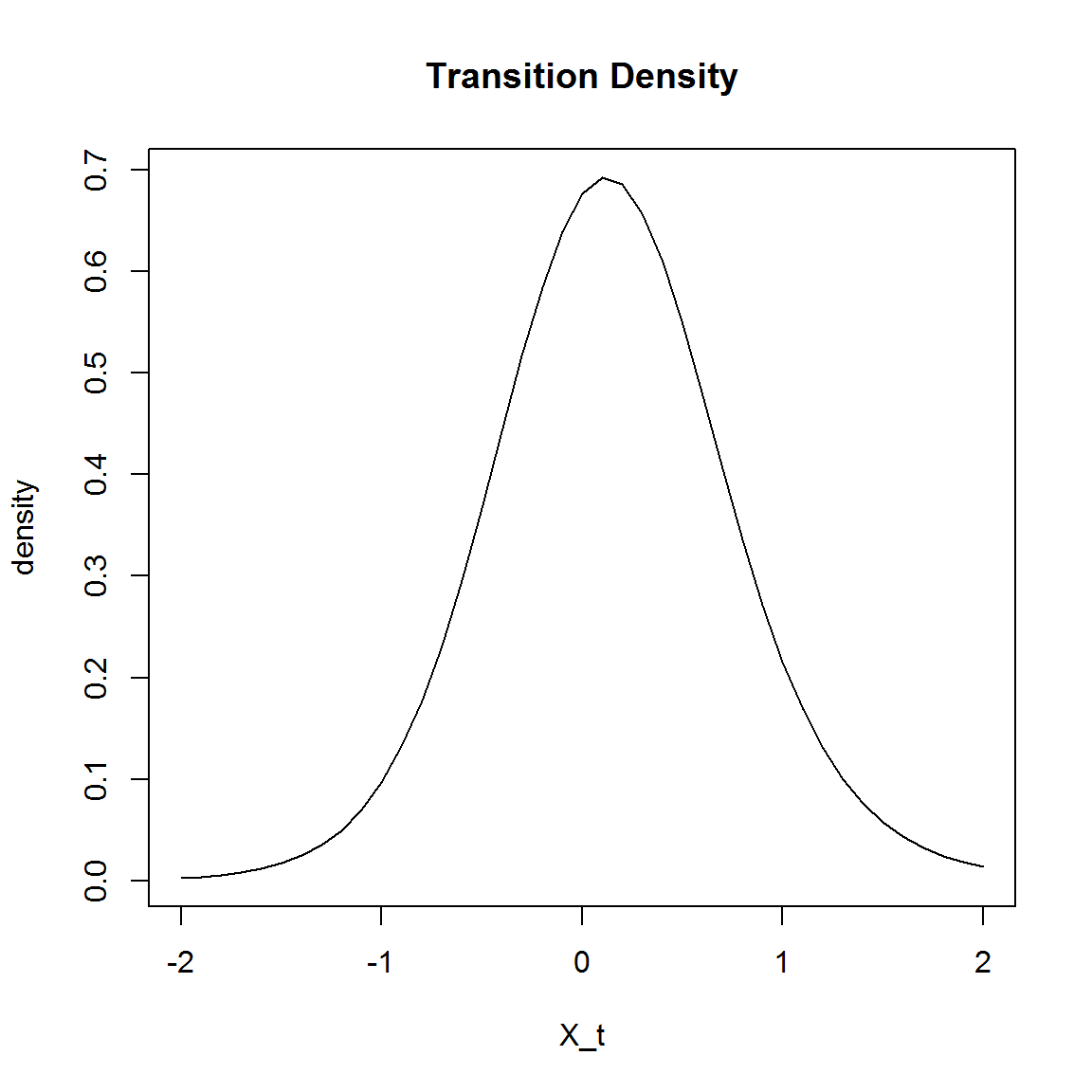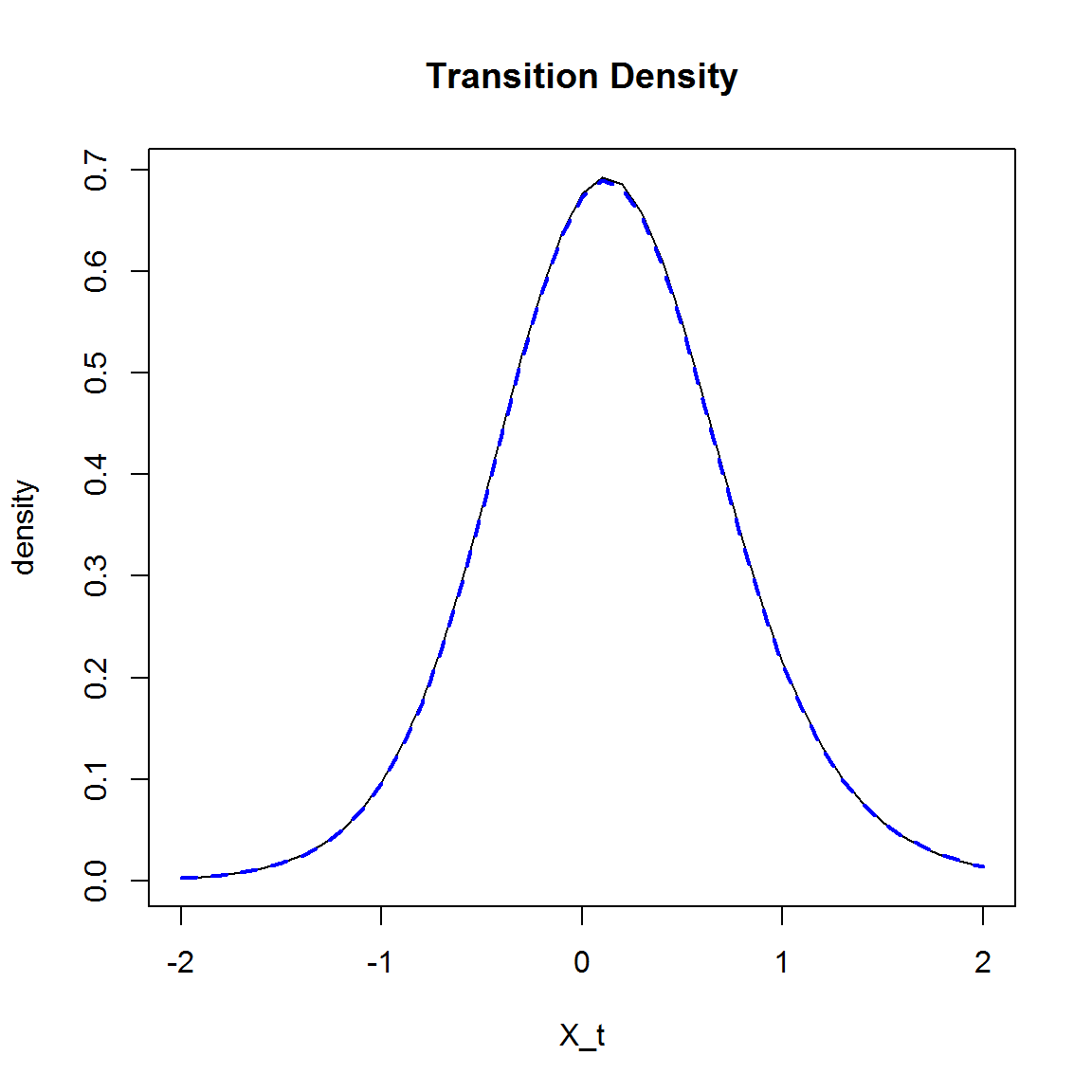# Introduction

DiffusionRjgqd is an R package for performing inference and analysis on Jump diffusion processes. Jump diffusion processes extend standard diffusion processes by allowing the trajectories of the process to undergo ‘shocks’ or ‘jumps’ at random points in time, inducing discontinuities in the trajectory of the process. As such, jump diffusion processes constitute an extremely useful class of mixture processes that enjoy a wide range of applications in various fields of science. As in the case of jump free diffusion processes, jump diffusion processes are difficult to work with since very few models have analytically tractable dynamics. For these purposes we have developed the DiffusionRjgqd package which extends upon the architecture DiffusionRgqd package in order to provide tools for performing analysis on a class of non-linear, time inhomogeneous jump diffusion processes with state-dependent jump mechanisms.

# Generalized quadratic jump diffusions (J-GQDs)

DiffusionRjgqd is built around a class of jump diffusion processes with quadratic drift and diffusion specifications and state dependent jump mechanisms. That is, for an SDE: $dX_t = \mu(X_t,t)dt +\sigma(X_t,t)dB_t +dP_t$ where $$B_t$$ is standard Brownian motion, the drift and diffusion terms assume at most second order non-linearity: $\mu(X_t,t) = G_0(t)+G_1(t)X_t+G_2(t)X_t^2$ and $\sigma^2(X_t,t) = Q_0(t)+Q_1(t)X_t+Q_2(t)X_t^2.$ In addition to being driven by Brownian motion, jump diffusions are driven by pure jump processes. Within the generalized quadratic jump diffusion framework the jump mechanism of the process is assumed to take the form: $dP_t = J(X_t,t,\dot{z}_t)dN_t$ for $J(X_t,t,\dot{z}_t) = \begin{cases} \dot{z}_t &\mbox{if jumps are additive}\\ \dot{z}_tX_t&\mbox{if jumps are multiplicative}\\ \end{cases}$ and $$N_t$$ is a counting process with arrival rate of the form: $\lambda(X_t,t) = \lambda_0(t)+\lambda_1(t)X_t+\lambda_2(t)X_t^2$

The purpose of this restriction is to provide a mathematical ‘sandbox’ within which we can calculate very accurate approximations to the transitional density of a diffusion process whilst maximizing freedom with respect to specifying time-inhomogeneous components. That is, within this framework, although we have limited the complexity of state-dependence in the drift and diffusion of the model, one has nearly complete freedom of specification for time-inhomogeneous coefficients of the model (i.e., G0(t), Q1(t) etc.).

# Interface

The interface of the DiffusionRjgqd package follows that of the related DiffusionRgqd package which is designed around a functional input system, where the user specifies the coefficients of the GQD as normal R-functions. For example, for a jump free diffusion with SDE:

$dX_t = -\sin(2\pi t)X_tdt +dB_t,$

the model can be written in R-syntax in terms of its GQD-coefficients as

G1 <- function(t){-sin(2*pi*t)}
Q0 <- function(t){1}

Building on this, if we add a jump component to the model, for example: $dX_t = -\sin(2\pi t)X_tdt +dB_t+dP_t$ with $dP_t = \dot{z}_tdN_t$ where $$\dot{z}_t\sim \mbox{N(0,0.5^2)}$$ and the counting process $$N_t$$ is subject to an arrival rate $$\lambda(X_t,t) = 0.15X_t$$, we can extend the model within the workspace by using the syntax:

G1   <- function(t){-sin(2*pi*t)}
Q0   <- function(t){1}
Lam1 <- function(t){0.15}
Jmu  <- function(t){0.0}
Jsig <- function(t){0.5} 

Once again, the idea is to provide a grammatical link between the lexical structure of a generalized jump diffusion model in written language, to the R language. Routines in the DiffusionRjgqd package thus operate by searching the current R workspace for pre-defined coefficient names after which it subsequently constructs the appropriate algorithm in order to perform analysis. For example, in the case of scalar jump-GQDs, recognized function names are tabled below.

Coefficient R-Syntax Coefficient R-Syntax
$$G_0(t)$$ G0=function(t){} $$Q_0(t)$$ Q0=function(t){}
$$G_1(t)$$ G1=function(t){} $$Q_1(t)$$ Q1=function(t){}
$$G_2(t)$$ G2=function(t){} $$Q_2(t)$$ Q2=function(t){}
Coefficient R-Syntax
$$\lambda_0(t)$$ Lam0=function(t){}
$$\lambda_1(t)$$ Lam1=function(t){}
$$\lambda_2(t)$$ Lam2=function(t){}
Jump Distribution Parameters R-Syntax
$$\mbox{N}(\mu_z(t),\sigma_z^2(t))$$ Location, scale Jmu(t), Jsig(t)
$$\mbox{Exp}(\lambda_z(t))$$ $$E(\dot{z}_t)=\lambda_z(t)$$ Jlam(t)
$$\mbox{Gamma}(\alpha_z(t),\beta_z(t))$$ Shape, scale Jalpha(t), Jbeta(t)
$$\mbox{Laplace}(a_z(t),b_z(t))$$ Location, scale Ja(t), Jb(t)
$$\mbox{Uniform}(a_z(t),b_z(t))$$ Support Ja(t), Jb(t)

# Brownian motion with drift and additive jumps

Consider a stochastic differential equation

$dX_t = \mu_x dt +\sigma_x dB_t+\dot{z}dN_t$

where $$B_t$$ is standard Brownian motion. Then the distribution of the process $$X_t$$ at time t, given that it started in state $$X_s$$ at time s with $$\dot{z}\sim \mbox{N}(\mu_z,\sigma_z^2)$$ and $$N_t-N_s \sim \mbox{Poi}(\lambda (t-s))$$, is given by (Yu 2007):

\begin{aligned} f(X_t|X_s)=&\frac{e^{-\lambda \tau}}{\sqrt{2\pi\sigma_x^2\tau}}\exp\bigg(-\frac{(X_t-X_s-\mu_x \tau)^2}{2\sigma_x^2 \tau}\bigg)\\&+\sum_{i=1}^\infty\frac{e^{-\lambda \tau}\lambda^i \tau^i}{i!}\frac{1}{\sqrt{2 \pi(\sigma_x^2\tau+i\sigma_{{z}}^2)}}\exp\bigg(-\frac{(X_t-X_s-\mu_x \tau-j\mu_{{z}})^2}{2(\sigma_x^2 \tau+i \sigma_{{z}}^2)}\bigg).\\ \end{aligned}

mu.x    <- 0.1
sigma.x <- 0.5
lam     <- 0.5
mu.z    <- 0.1
sigma.z <- 0.5

true.density=function(X0,Xt,t,order =10)
{
dens <- exp(-lam*t)*dnorm(Xt,X0+mu.x*t,sqrt(sigma.x^2*t))
for(i in 1:order)
{
dens <-  dens +exp(-lam*t)*(lam*t)^i/factorial(i)*dnorm(Xt,X0+mu.x*t+i*mu.z*t,sqrt(sigma.x^2*t+i*sigma.z^2*t))
}
return(list(density=dens,Xt=Xt))
}
Xt  <- seq(-2,2,1/10)
res <- true.density(0,Xt,1)
plot(res$density~Xt,type='l',main='Transition Density',xlab='X_t',ylab='density')Using DiffusionRjgqd we can replicate the transition density of the above model by using the GQD-framework. This is achieved by defining the model in terms of its coefficients as per the GQD-framework. In R, for example, we can use the JGQD.density() function in order to generate the transitional density (if the interface seems a bit strange, have a look at the vignettes and package paper of the DiffusionRgqd). library(DiffusionRjgqd) # Remove any existing coefficients: JGQD.remove() ##  "Removed : NA " # Define the model coefficients: G0 <- function(t){mu.x} Q0 <- function(t){sigma.x^2} Lam0 <- function(t){lam} Jmu <- function(t){mu.z} Jsig <- function(t){sigma.z} # Calculate the transitional density: BM <- JGQD.density(0,Xt,0,1,factorize=TRUE,Dtype='Normal.A') ## ## ================================================================ ## Jump Generalized Quadratic Diffusion (JGQD) ## ================================================================ ## _____________________ Drift Coefficients _______________________ ## G0 : mu.x ## G1 ## G2 ## ___________________ Diffusion Coefficients _____________________ ## Q0 : sigma.x^2 ## Q1 ## Q2 ## _______________________ Jump Mechanism _________________________ ## ......................... Intensity ............................ ## Lam0 : lam ## Lam1 ## Lam2 ## ........................... Jumps .............................. ## Normal ## Jmu : mu.z ## Jsig : sigma.z ## __________________ Distribution Approximant ____________________ ## Density approx. : Normal.A ## Trunc. Order : 8 ## Dens. Order : 4 ## ================================================================= # Plot the transitional density: plot(res$density~Xt,type='l',main='Transition Density',xlab='X_t',ylab='density')
lines(BM$density[,100]~BM$Xt,col='blue',lty='dashed',lwd=2)browseVignettes('DiffusionRjgqd')